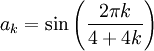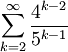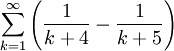# Quiz 1

Previous: Quizzes

Next: Quiz 2

The following quiz covers topics in Unit 1.

If you would like to know how well you did on the quiz, you can have the quiz results sent to an email address that you provide at the end of the quiz. You will also be sent partial solutions.

## Quiz 1: Infinite Sequences and Series

Progress:

Determine whether the infinite sequenceconverges. If it does, what does the sequence converge to?

What is the value ofWhat is the value ofExpress 0.571571571571 ... as a ratio of two integers.

Determine whether the infinite sequenceconverges. If it does, what does the sequence converge to?

### Submit

Previous: Quizzes

Next: Quiz 2